Fractions

# 10 Dividing Fractions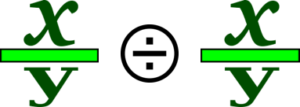Dividing fractions follows similar logic to multiplying fractions. It involves working with the numerators and denominators separately. Once you have done your initial calculations, you put it all together to get your answer. There is a little twist, though, that we’ll have to examine as we work through some questions.

Before we get to all that, let’s start by revisiting dividing whole numbers, and then we’ll work our way up to fractions.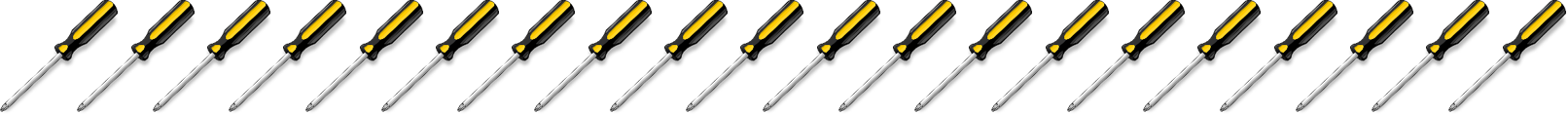Now divide those 20 screwdrivers by 10 (or into groups of 10).

$\LARGE20÷10=2$

You end up with 2 groups of 10.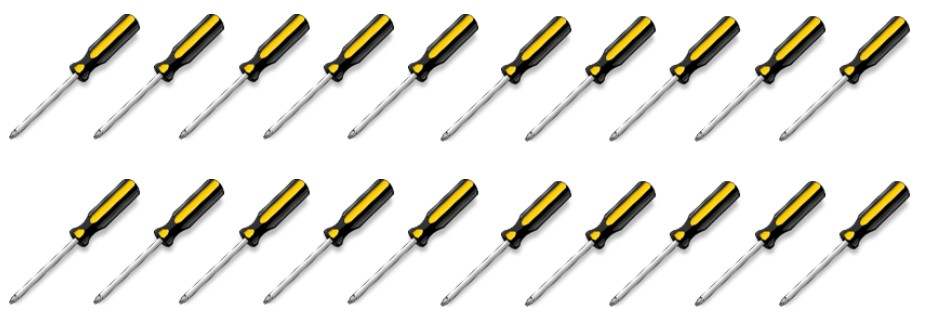Now divide those 20 screwdrivers by 5 (or into groups of 5).

$\LARGE20÷5=4$

You end up with 4 groups of 5.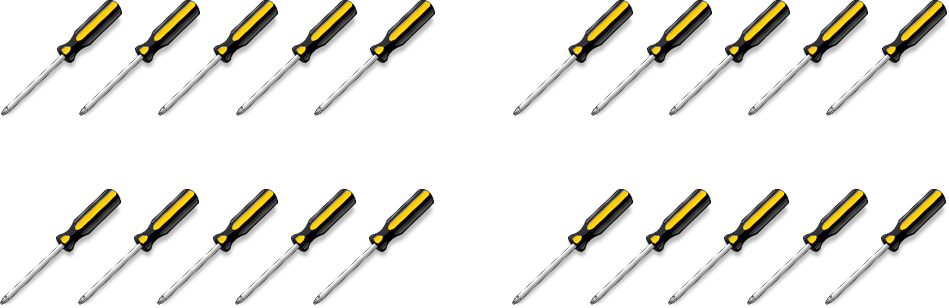Now divide those 20 screwdrivers by 2 (or into groups of 2).

$\LARGE20÷2=10$

You end up with 10 groups of 2.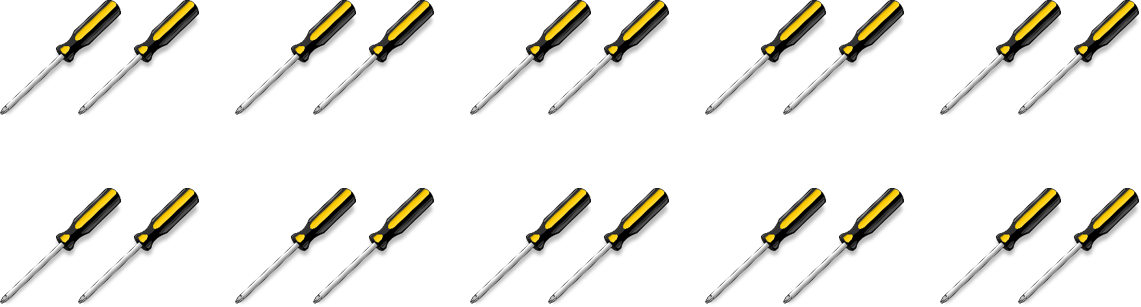Take a look at the math here. Do you see a pattern? What did you come up with? Did you notice that, when you take your original amount (in this case, 20) and divide it by a number that continues to get smaller (10, then 5, then 2), we end up with an answer that gets larger.

$\LARGE20÷10=2$

$\LARGE20÷5=4$

$\LARGE20÷2=10$

Follow this logic into fractions, keeping in mind that fractions are not only less than 10, 5, and 2, but 1. Using this pattern, we determine that dividing the 20 screwdrivers by a number less than 1 would get us a larger answer than if we divided the 20 by 10, 5, or 2.

Try this. Take the 20 screwdrivers, and divide them by ½. What do you think your answer will be?

$\LARGE20÷\dfrac{1}{2}=?$

Following our logic, the answer should be more than 10, and in fact, it is.

$\LARGE20÷\dfrac{1}{2}=40$

This does not mean that we end up with 40 screwdrivers, though. What it means is that we end up with 40 parts of screwdrivers. You have to imagine that each of the screwdrivers were split into 2. Twenty screwdrivers split in half would give us 40 pieces in the end. The question now becomes, how do we do this mathematically? The answer lies in using what is known as a reciprocal. Here is the definition.

Reciprocal: A number that has a relationship with another number such that their product is 1.

This means that, when you take a number such as 5 and then multiply it by its reciprocal, you will end up with an answer of 1. We’ll start with the whole number 5. We could also write the number 5 as a fraction.

$\LARGE5=\dfrac{5}{1}$

Using our definition of reciprocal, we need to find a number that, when multiplied by 5/1, gives us an answer of 1.To find the answer, we have to go back to multiplying fractions. Remember that, when we multiply fractions, we just multiply the numerators together and then multiply the denominators together. From this, we can conclude that:

$\LARGE\dfrac{5}{1}\times\dfrac{1}{5}=1$

In the end, to find the reciprocal of a fraction, we simply take the numerator and make it the denominator and take the denominator and make it the numerator. Essentially, we are just flipping the fraction around. Here are some more examples of reciprocals.

$\LARGE\dfrac{3}{8}\text{ and }\dfrac{8}{3}$

$\LARGE\dfrac{2}{9}\text{ and }\dfrac{9}{2}$

$\LARGE\dfrac{24}{17}\text{ and }\dfrac{17}{24}$

Okay, so now that we have the reciprocal issue out of the way, the question then becomes, why do we need reciprocals in the first place? Well, the answer lies in the rule for dividing fractions.The rule for dividing fractions is you take the first fraction and multiply it by the reciprocal of the second fraction. Yes, you heard that right: to divide, you end up multiplying, but only after first flipping the second fraction around.

Flipping the second fraction around (finding its reciprocal) changes the value of the equation. In order to keep the equation mathematically the same, we have to change the division question into a multiplication question. Take a look at the following example to see how this is done.

Example

$\LARGE\dfrac{1}{2}÷\dfrac{3}{8}=?$

Step 1: Put the question into a form that you can work with. This involves finding the reciprocal of the second fraction and then multiplying it by the first.

$\LARGE\text{The reciprocal of }\dfrac{3}{8}\text{ is }\dfrac{8}{3}$

$\LARGE\text{Check: }\dfrac{3}{8}\times\dfrac{8}{3}=\dfrac{24}{24}=1$

So we end up with:

$\LARGE\dfrac{1}{2}÷\dfrac{3}{8}\text{ becomes }\dfrac{1}{2}\times\dfrac{8}{3}=?$

Step 2: Follow the same procedure as we did when multiplying fractions. Multiply the numerators together and then multiply the denominators together.

Multiply the numerators together

$\LARGE1\times8=8$

Multiply the denominators together

$\LARGE2\times3=6$

Step 3: Take these answers and put them back into a fraction.

$\LARGE\dfrac{1}{2}\times\dfrac{8}{3}=\dfrac{8}{6}$

Step 4: Put the answer into lowest terms and then into a mixed number, if necessary.$\LARGE\dfrac{4}{3}=1\dfrac{1}{3}\text{ Mixed number}$

$\LARGE\dfrac{1}{2}÷\dfrac{3}{8}=1\dfrac{1}{3}$

Example

$\LARGE\dfrac{5}{9}÷\dfrac{7}{4}=?$

Step 1: Put the question into a form that you can work with. This involves finding the reciprocal of the second fraction and then multiplying it by the first.

$\LARGE\dfrac{5}{9}÷\dfrac{7}{4}\text{ becomes }\dfrac{5}{9}\times\dfrac{4}{7}$

Step 2: Multiply the numerators together and then multiply the denominators together.

Multiply numerators together

$\LARGE5\times4=20$

Multiply denominators together

$\LARGE9\times7=63$

Step 3: Take these answers and put them back into a fraction.

$\LARGE\dfrac{5}{9}\times\dfrac{4}{7}=\dfrac{20}{63}$

Step 4: Put the answer into lowest terms and then into a mixed number, if necessary. In this case, the answer is both in lowest terms and is a proper fraction already, so we are done.

$\LARGE\dfrac{5}{9}÷\dfrac{7}{4}=\dfrac{20}{63}$

# Practice Questions

Try these practice questions and take a look at the video answers to see how you did.

Question 1

$\LARGE\dfrac{7}{8}÷\dfrac{7}{16}=$

Question 2

$\LARGE4\dfrac{7}{8}÷2\dfrac{3}{4}=$

Note: This one is slightly different from what we have been doing, as it involves dividing mixed numbers. What do you think you are going to have to do when dealing with this?

ANSWER: You have to change the mixed number into an improper fraction first. Then you can work through the question the same way we have been doing before.

https://video.bccampus.ca/id/0_q09vuaii?width=608&height=402&playerId=23448552